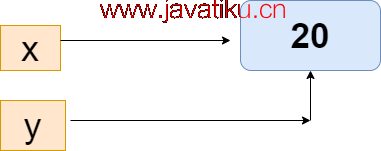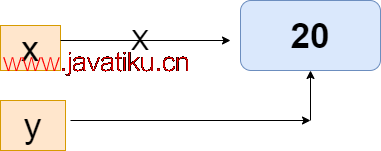# Tkinter 教程-Python 内存管理## Python 内存分配

• 静态内存分配
• 动态内存分配

### 静态内存分配 -

``static int a=10;``

### 动态内存分配

``````int *a;
p = new int;  ``````

## 默认的 Python 实现

Python 是一种开源、面向对象的编程语言，其默认实现是使用 C 编程语言编写的。这是一个非常有趣的事实 - 一种最流行的语言是用另一种语言编写的？但这并不完全是真的，但有点类似。

## Python 内存中的对象

``````a= 10
print(a)
del a
print(a)``````

``````10
Traceback (most recent call last):
File "", line 1, in
print(x)
NameError : name 'a' is not defined``````

## Python 中的引用计数

``x = 20  ````````x = 20
y = x
if id(x) == id(y):
print("The variables x and y are referring  to the same object")  ````````````x = 20
y = x
x += 1
If id(x) == id(y):
print("x and y do not refer to  the same object")``````

``x and y do not refer to the same object``

## 调整垃圾收集器

Python 垃圾收集器通过其生成对对象进行分类。Python 垃圾收集器有三代。当我们在程序中定义新对象时，垃圾收集器的第一代处理它的生命周期。如果对象在不同程序中有用，它将被提升到下一代。每一代都有一个阈值。

``````Import GC
print(GC.get_threshold())``````

``(700, 10, 10)``

``````import gc
gc.set_threshold(800, 20, 20) ``````

Python 垃圾收集器处理开发人员的低级细节。

## 手动执行垃圾收集的重要性

``````def cycle_create():
list1 = [18, 29, 15]
list1.append(list1)
return list1

cycle_create()
[18, 29, 15, [...]]  ``````

``````import gc
n = gc.collect()
print("Number of object:", n) ``````

gc.collect() 方法用于执行基于时间的垃圾收集。此方法在固定时间间隔后调用以执行基于时间的垃圾收集。

``````import sys, gc

def cycle_create():
list1 = [18, 29, 15]
list1.append(list1)

def main():
print("Here we are creating garbage...")
for i in range(10):
cycle_create()

print("Collecting the object...")
num = gc.collect()
print("Number of unreachable objects collected by GC:", num)
print("Uncollectable garbage:", gc.garbage)

if __name__ == "__main__":
main()
sys.exit()  ``````

``````Here, we are creating garbage...
Collecting the object...
Number of unreachable objects collected by GC: 10
Uncollectable garbage: []``````

## CPython 内存管理

Python 使用内存的一部分用于内部使用和非对象内存。另一部分内存用于 Python 对象，例如 int、dict、list 等。

CPython 包含了分配对象的对象分配器。对象分配器每次新对象需要空间时都会得到调用。分配器主要用于小数据量，因为 Python 不会一次处理太多数据。只有在绝对需要时才会分配内存。

CPython 内存分配策略有三个主要组成部分。

Arena - 这是内存中最大的块，并且在内存中对齐到页边界。操作系统使用页边界，这是一块固定长度的连续内存块的边缘。Python 假设系统的页大小为 256 千字节。

Pools - 它由单个大小类组成。相同大小类的池管理一个双链列表。池可以是已使用、已满或空的。一个 已使用 的池包含用于存储数据的内存块。一个 已满 的池包含所有已分配的内存块并包含数据。一个空池没有任何数据，并且可以在需要时为块分配任何大小类。

• 避免列表切片

• 谨慎使用列表索引

• 字符串连接

• 避免不必要的全局变量

• 使用列表推导式